# A powerful method for explainability of Object Detection Algorithms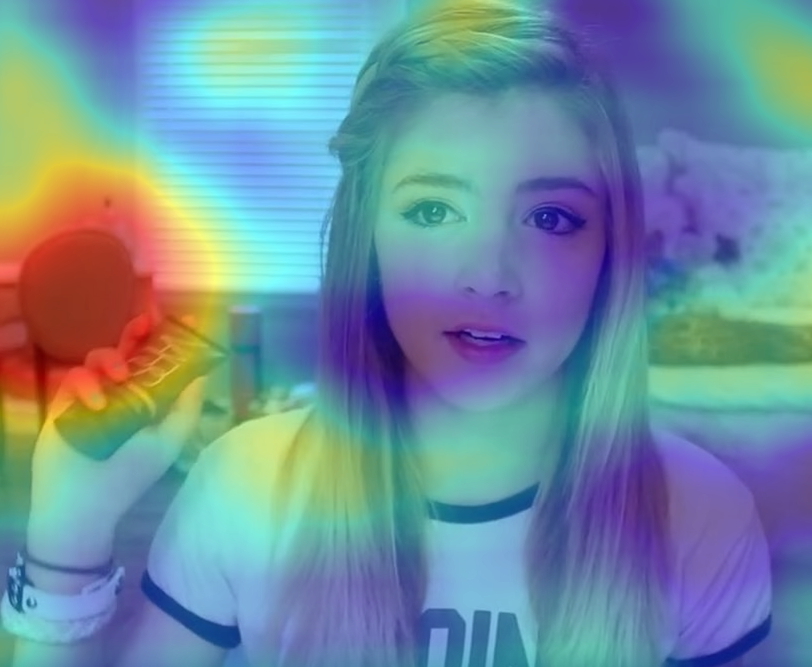Heatmap for a Yolo prediction

Since the R-CNN Object Detection (OD) was released in 2014 until now, deep learning approaches to object detection problems are becoming more and more popular.

OD is now used in almost every field from agriculture to autonomous driving. But one of the main disadvantages of the OD techniques is the lack of interpretability of its outputs that are not human-readable and this is a big concern.

Since that prediction without interpretable explanation will have restricted applicability eventually.

Until recently the only way we could measure how good the outcome of a model is was to evaluate its predictions using a validation dataset, which is a dataset that has images not used to train the model.

But the only thing that we can assure when a model has good performance metrics when applied to a validation dataset is that the model learned to detect the image scenarios of the validation dataset. But if the validation dataset does not have all the possible real-life scenarios, problems can happen.

There are some famous examples when the deep learning models were having amazing results at the training and validation data, but because its results are not human-readable the model was predicting something completely different from this goal.

The most famous example is from the military that trained a model with 100% accuracy to predict tanks that had a poor result in the real-life.

That result occurred because at the training and validation dataset all American tanks had been photographed on a sunny day and all the Russian tanks had been photographed on a cloudy day.

So the model instead of detecting patterns at the tanks was detecting patterns of the sky.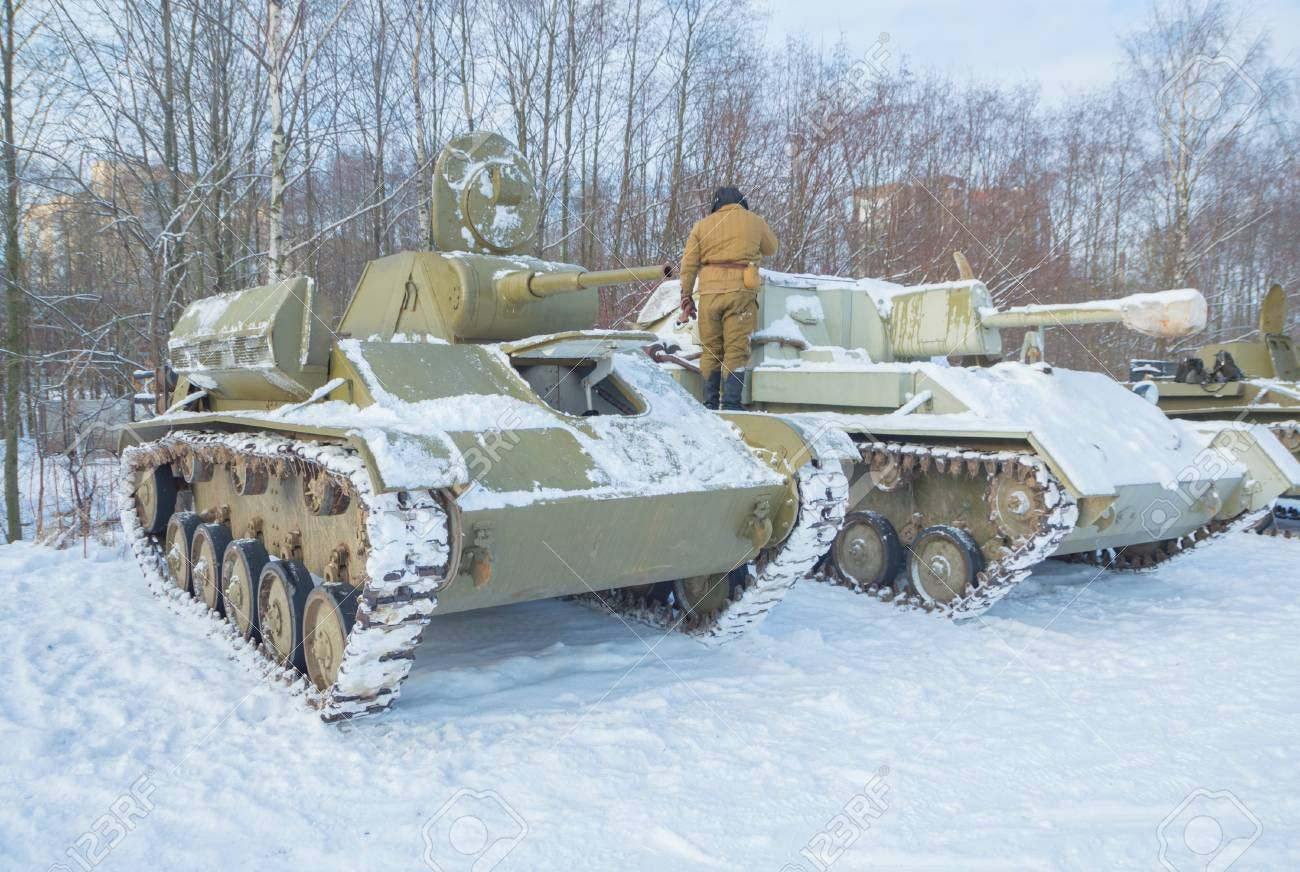Tank on a sunny day and tanks on a cloudy day

To help the team at having higher reliability that a deep learning model was ready for production a new technique was built using a heatmap to show the areas of an image that the model was giving higher importance to generate the outcome.

First, the heatmaps started to be used at classification tasks, then some methods have emerged to object detection models like the one used for the Single Shot Detector (SSD).

But that explainability techniques are not so intuitive and can not be reproduced at other architectures, even though are usually fast.

Finally, in June 2020 Vitali Petsiuk published the Black-box Explanation of Object Detectors via Saliency Maps that is an agnostic approach for generating visual explanations for the predictions of any OD model.

The D-Rise technique shown in the article facilitated the creation of heatmaps because with just one implementation the user can create heatmaps for any OD model like Retinanet, SSD, Yolo, etc…

Randomized Input Sampling for Explanation (RISE) is a technique that uses randomly masked versions of the input image to generate heatmap representations of Classification models.

D-RISE is inspired by the RISE method but designed to address the OD problem where the output is more complex, composed by a list of bounding box coordinates, the array of probabilities, and some algorithms have also the objectness score.

At D-RISE first, you get the bounding box of the original image, then you apply a random noise called mask to N images, pass the masked images to the model and compare the output of the masked images with the output from the original image to get the heatmap.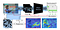D-RISE technique summarized

D-RISE, a black-box attribution technique for explaining object detectors via saliency maps, by significantly extending the idea of exhaustively and systematically exploring areas that affect the prediction outputs of the underlying model by manipulating its inputs.

First, you have to generate N RISE masks following the steps from the paper:

1. Sample N binary masks of size h × w (smaller than image size H × W ) by setting each element independently to 1 with probability p and to 0 with the remaining probability.
2. Upsample all masks to size (h+1)CH×(w+1)CW using bilinear interpolation, where CH × CW = ⌊H/h⌋ × ⌊W/w⌋ is the size of the cell in the upsampled mask.
3. Crop areas H × W with uniformly random offsets ranging from (0, 0) up to (CH, CW).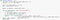Python code to generate the masks from https://github.com/eclique/RISE/blob/master/Easy_start.ipynb

After that, you only need to have a Numpy array representation of the original image resized to the size of the mask and multiply that array by the mask array taking advantage of vectorization.

The result of this step has the shape N × H × W and is represented by M.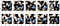Example of masked images

Second, pass the original image as input to the model to get its output. You can create a BoundingBox class to make the code more readable having the attributes:

1. Bounding box coordinates (xmin, ymin, xmax, ymax) are called Li in the article.
2. The Objectness score, the probability that this box contains an object of any type called Oi.
3. The list of probabilities for each one of the Classes is called Pi.

Or you can use only np.arrays.

So at the second step, you will have a list of bounding boxes objects predicted by the model that the article called Dt having the shape T ×(4+1+C) where:

• T is the number of predicted bounding boxes
• 4 are the four coordinates of the box (Li)
• 1 is the Objectness score (Oi)
• C is the list of probabilities (Pi)

Third, get all your masked images and repeat the second step, here the results are called Dp and will have the shape N ×Np ×(4+1+C), where N is the number of masks, Np is the number of boxes per mask and the others are the same from the step above.

Fourth compute pairwise similarities between two sets of detection vectors Dt and Dp and take the maximum score per each masked image per each target vector.

So to each list of bounding boxes at Dp, you are going to calculate the following similarity score (s) to each box object at Dt and take the maximum score per each masked image per each box of Dt.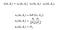Similarity metric

Where sl is the intersection over union metric (IoU) and sp is the cosine distance between the classes probabilities. The result of this step has the shape N × T and is represented by Wit.

The last step is to compute a weighted sum of masks Mi concerning computed weights Wit to get the saliency maps.

S has the shape T × H × W.

Finally, you have the Saliency map for each one of the predicted objects of the original image, now you just need to overlay those results with the image to get a nice heatmap representation.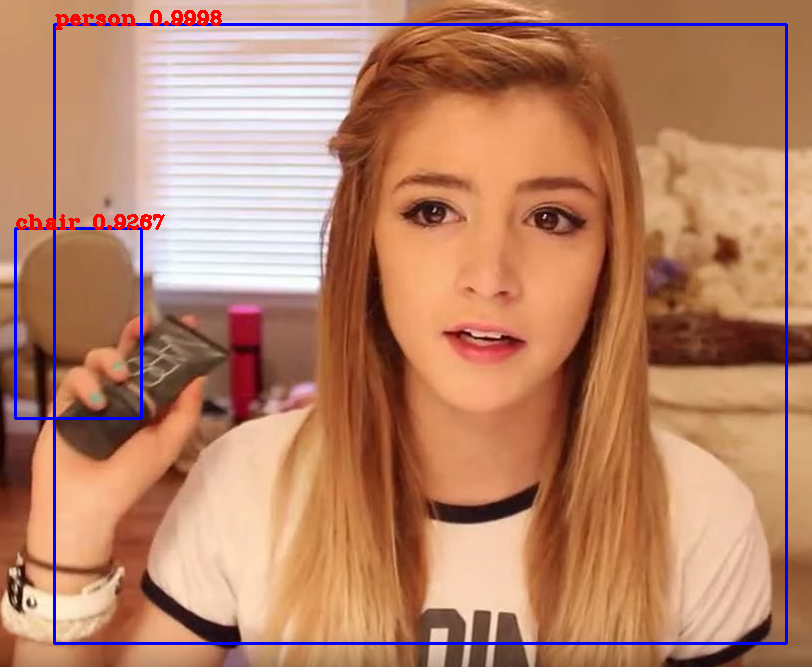Prediction for a yolo model trained at COCO dataset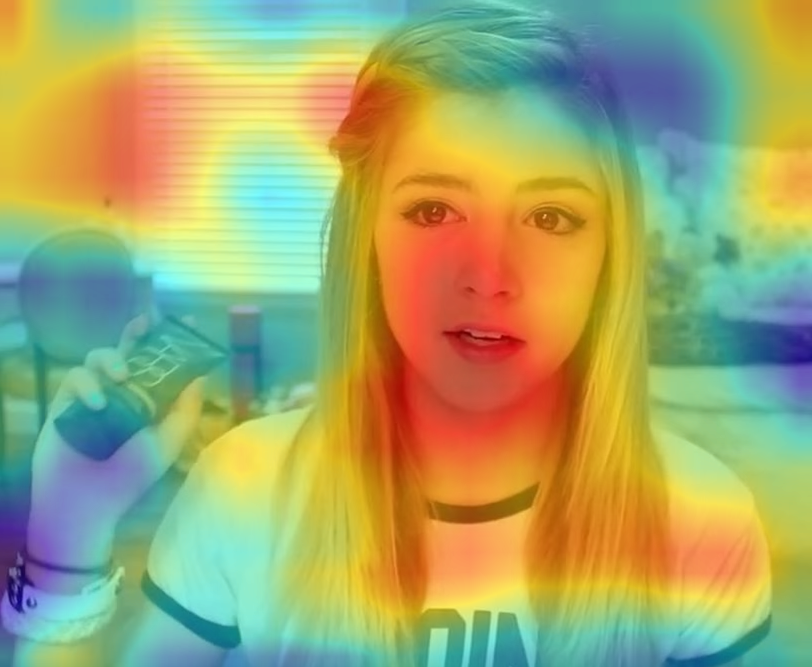Heatmap for the chair prediction using 1000 maks and heatmap for the person using 100 masks

As you can see in the images above the model predicted correctly the chair and the person. At the heatmap, the hotter the color the bigger the importance of that pixel to the model output and we could see that the model gave more importance to the chair and to the girl’s face indeed.

The parameters that you can tune to have better heatmaps representation are the parameters from the mask generation, at the heatmaps image we can see that the heatmap generated using 1000 masks has less noise than the heatmap generated using 100 maks.

So to your problem domain, you can test the number and size of the masks that best suits your needs. In the paper, the author used 5000 masks with size (16, 16).

One simple test that you can do is to train the model with a low number of epochs (a number that makes the model not converge) and then generate the heatmap.

The heatmaps of this poor model shall produce unintelligible heatmaps like the ones below.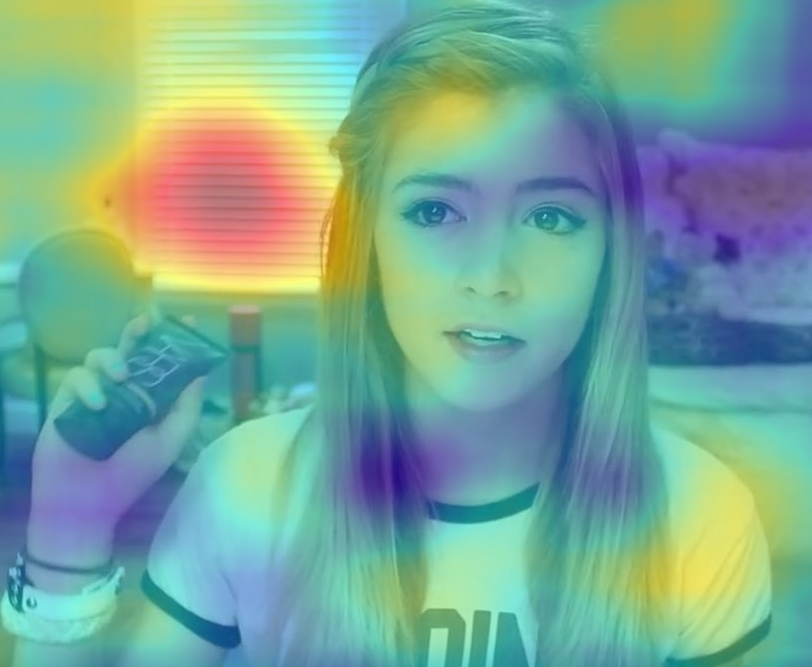Poor model heatmaps

A more robust metric to evaluate the heatmap quality is the deletion and insertion metrics proposed in the RISE paper. I got the step-by-step made by the authors of the article.

For a classification task, the deletion metric measures the drop in class probability as more and more pixels are removed in the order of decreasing importance.

Intuitively it evaluates how well the saliency map represents the cause for the prediction. If the probability drops fast and its chart is steep, then the pixels that were assigned the most saliency are indeed important to the model.

The metric reports the area under the curve (AUC) of the probability vs. fraction of pixels removed as the scalar measure for evaluation. Lower AUC scores mean steeper drops in similarity, and therefore are better.

Insertion is a symmetric metric that measures the increase in probability while inserting pixels into an empty image. Higher AUC is better for insertion.

As you can imagine, this process is not fast. At the paper setup, D-RISE runs in approximately 70s per image for YOLOv3 and 170s for Faster R-CNN, on NVidia Tesla V100.

We still don’t know why the model chose the areas in hotter colors as more important to make the prediction.

In this article, we saw that it is possible to create an understandable, interpretable outcome of Deep Learning Models, that despite being slow it is the only agnostic way to create heatmaps for OD algorithms.

How the D-RISE technique works, the step-by-step to reproduce it at an OD model, and some methods that you can do to validate the heatmaps.

Unfortunately, I used the D-RISE code at work, and because of confidentiality I can’t share it, but I am sure that by reading this medium post and the original paper you can easily implement this method!

Yolo model used code https://github.com/zzh8829/yolov3-tf2

# Acknowledgments

• Paper authors: Vitali Petsiuk, Rajiv Jain, Varun Manjunatha, Vlad I. Morariu, Ashutosh Mehra, Vicente Ordonez, and Kate Saenko.

If you like what you read be sure to 👏 it below and share with your friends. I would be very happy and is free for you!

## More from Jair Neto

https://www.linkedin.com/in/jair-guedes-ferreira-neto/